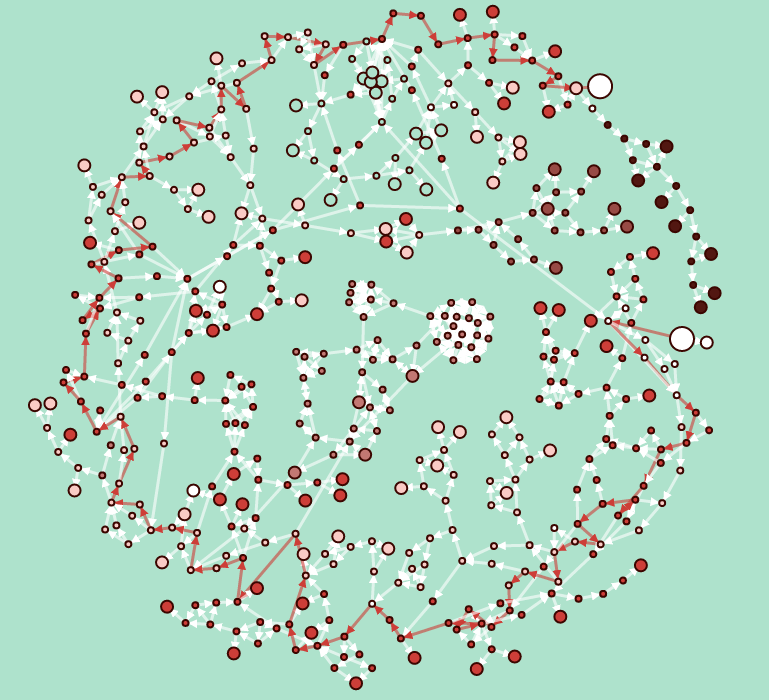# Romeo and/or Juliet and/or Graph Theory

## and/or Data Processing

Posted by Dave Anderson on July 22, 2016

I recently completed a visualization of passages and choices in Ryan North’s excellent chooseable path adventure, Romeo and/or Juliet.This is the second part of a series of posts about some of lessons learned and the technical details from how I put this together. Read the others about book impressions, data entry and data visualization as well.

# Data Processing Fun Times

Python’s Networkx library provides a very robust data structure for storing and transforming graph data. I’ve simplified the code below to highlight only the relevant Networkx code, but the full code is available on GitHub. Check it out!

Initializing our empty Digraph is straightforward.

``````G = nx.DiGraph()
``````

For each of the passages, adding the graph node and its properties is easy as well.

``````G.add_node(passage,
description=description,
pov=pov,
is_ending=is_ending,
tags=tags)
``````

Adding the choice data for building the graph edges is simple too.

``````G.add_edge(from_passage, to_passage,
description=description,
bard_choice=bard_choice,
weight=weight)
``````

The code to export the finished graph as JSON formatted node link data is just as simple.

``````d = json_graph.node_link_data(self.G)
json.dump(d, open(json_file, 'w'))
``````

# Graph Theory Fun Times

The Networkx library also makes it very easy to calculate classic graph algorithms against your data set and add the results as parameters to the node or edge. I played with using the class unweighted shortest path algorithm and Dijrska’s algorithm for weighted shortest path.

## Path of least decisions

By calculating the unweighted shortest path for each node, starting from the Cover of the story, we can easily add the shortest path length and shortest path passages visited to our graph node properties.

``````def add_shortest_path(self, start='Cover'):
# calculate min_depth from shortest path from 'Cover'
spl = nx.shortest_path_length(self.G, start)
nx.set_node_attributes(self.G, 'from_{}_length'.format(start.lower()), spl)

sp = nx.shortest_path(self.G, start)
nx.set_node_attributes(self.G, 'from_{}_path'.format(start.lower()), sp)
``````

This allows us to quickly do queries such as `raoj.G.node['THE END FOR REAL THIS TIME']['from_cover_path']` to find that least number of decisions to get from the Cover to the true ending of the story is 49 passages, quite a bit shorter than the 85 steps needed to follow the full bard path.

``````['Cover', '1', '36', '4', '5', '6', '7', '8', '9', '10', '25', '33', '58', '74', '96', '111', '120', '148', '167', '110', '142', '170', '158', '178', '149', '156', '199', '193', '216', '209', '191', '201', '189', '210', '221', '229', '258', '358', '365', '292', '332', '314', '329', '347', '355', '381', '425', '414', '434', '461', 'THE END FOR REAL THIS TIME']
``````

## Path of Least Page Turns

The last fun property that I played with adding was the “laziest path” a weighted shortest path from the cover to any node, where the weight for any choice was how many passages you would need to turn over in order to get to your choice.

We define the weight before adding the edge (choice) data.

``````try:
weight = abs(int(from_passage) - int(to_passage))
except:
weight = 1
``````

Adding the shortest weighted path is basically the same as the previous ccode but we are using Dijkstra’s algorithm instead the unweighted shortest path.

``````  spl = nx.dijkstra_path(self.G, start, end)
``````

We can query our results in the same manner as we saw earlier. This path is a little bit longer than our shortest path with 51 passages read, but with 888 passages turned over it is considerably more lazy than the 1010 passages turned over in the unweighted shortest path.

``````['Cover', '1', '36', '4', '5', '6', '7', '8', '9', '10', '25', '33', '58', '74', '96', '111', '120', '154', '144', '159', '139', '163', '122', '169', '143', '160', '172', '184', '193', '216', '209', '191', '201', '189', '210', '221', '229', '245', '268', '281', '311', '292', '332', '314', '329', '347', '355', '381', '425', '414', '434', '461', 'THE END FOR REAL THIS TIME']
``````

It might be fun to add these shortest/laziest paths to the graph visualization in the future through a REST web service with caching, but for now, we just have passages and choices, which is kinda awesome too.

The next part of this series discusses the aspects of doing layout and visualization for passages.